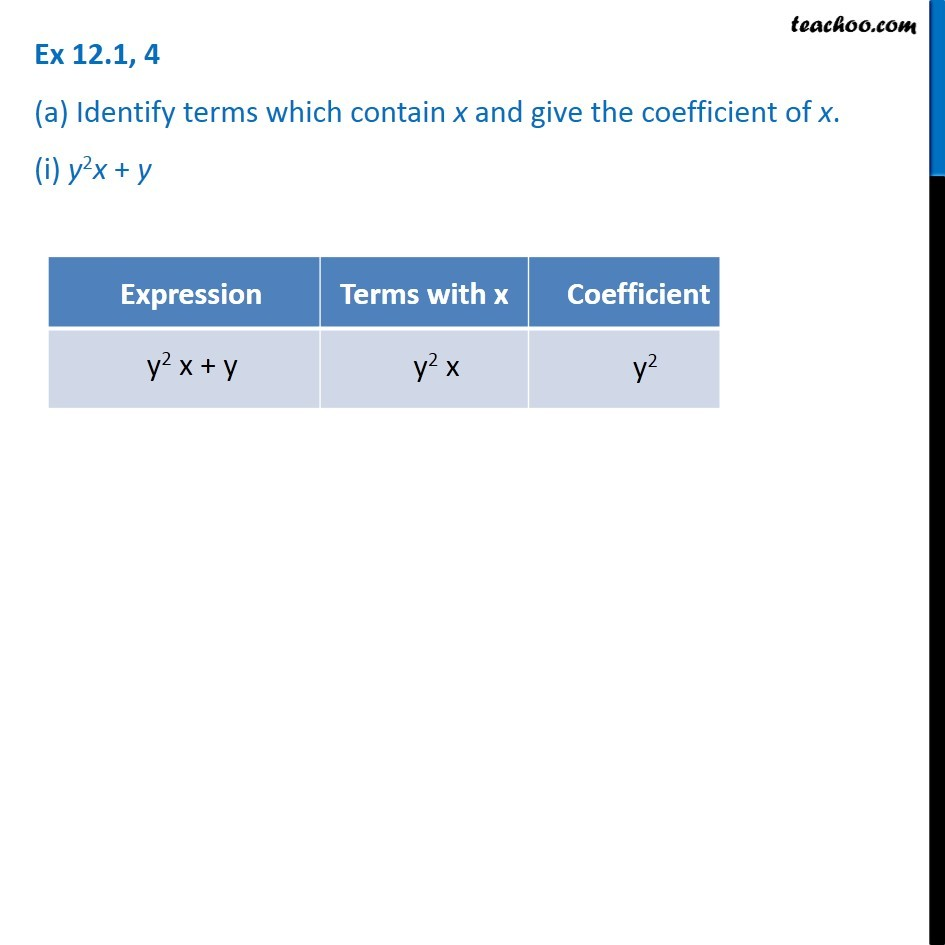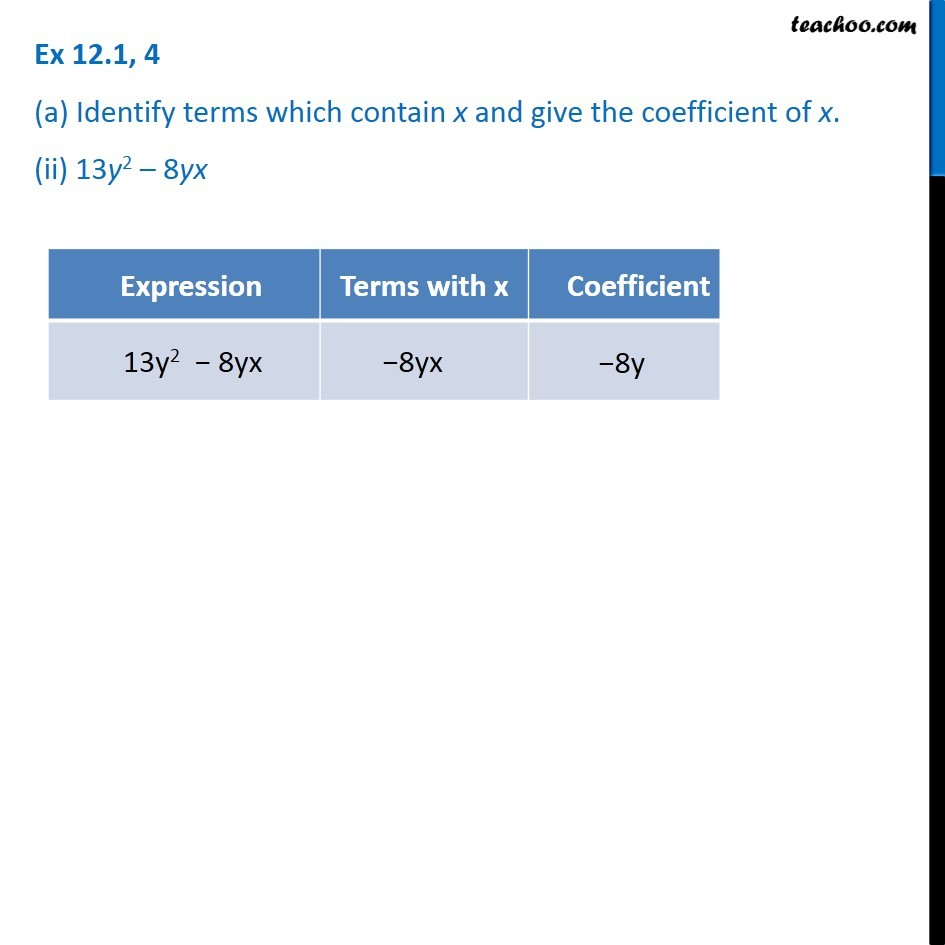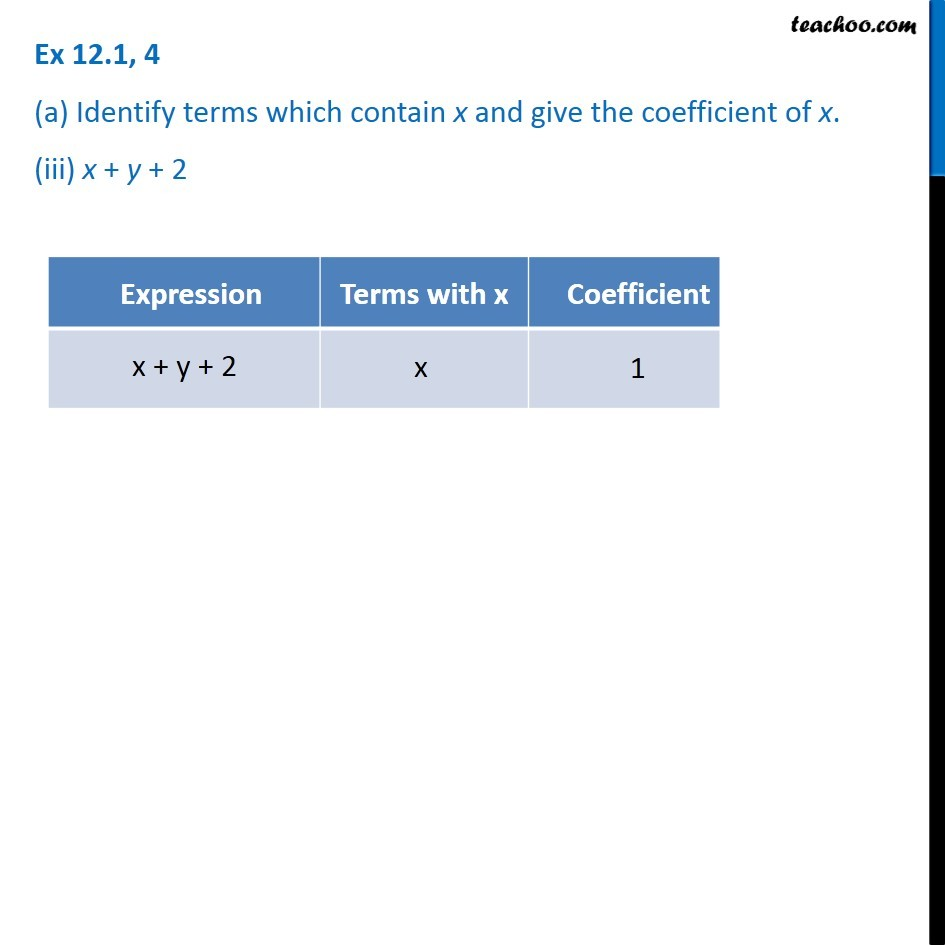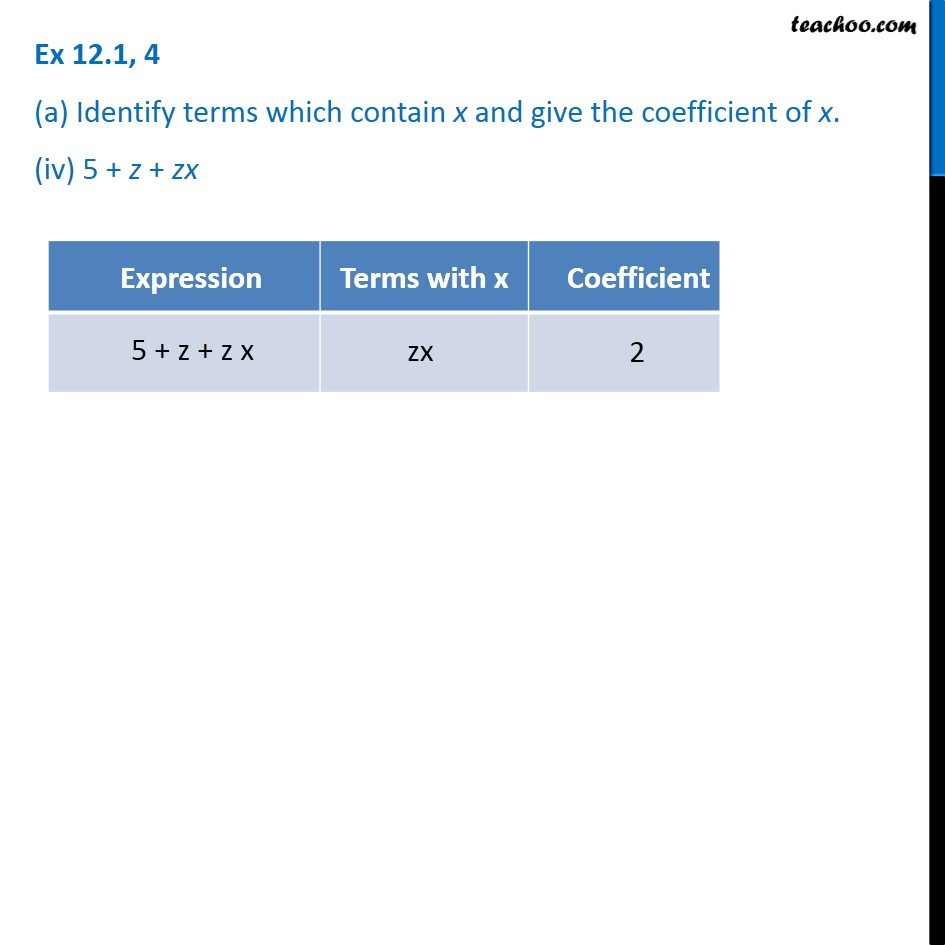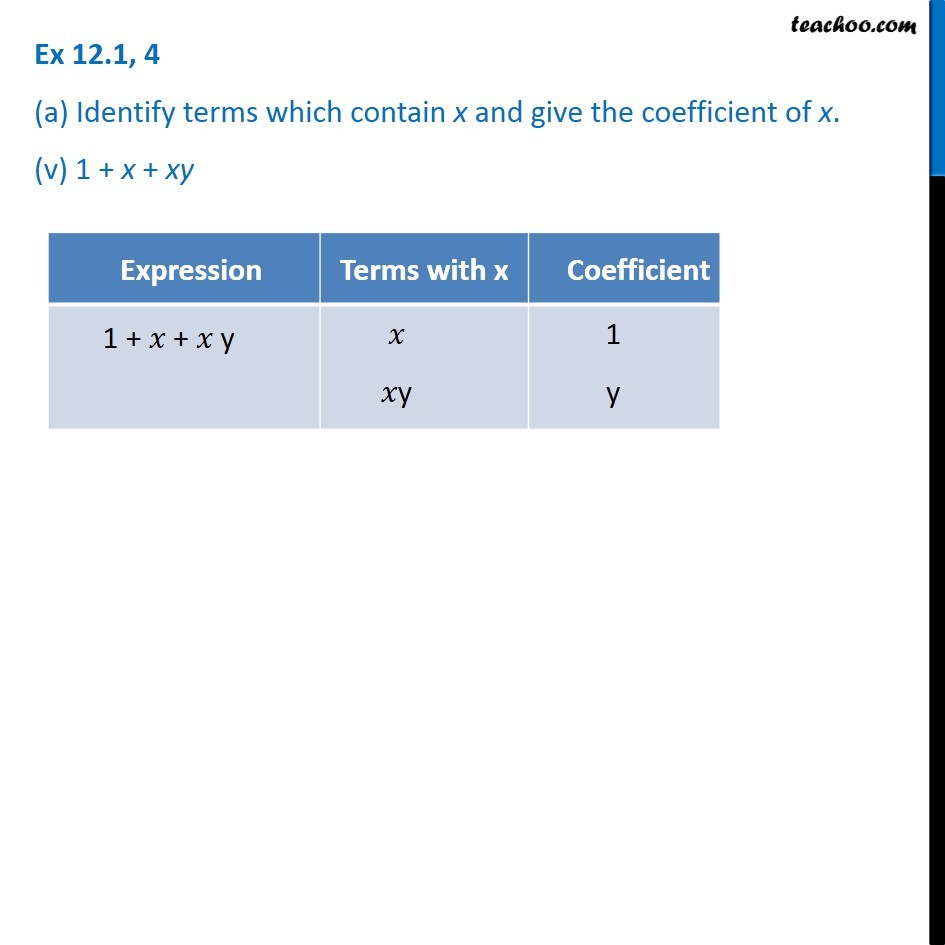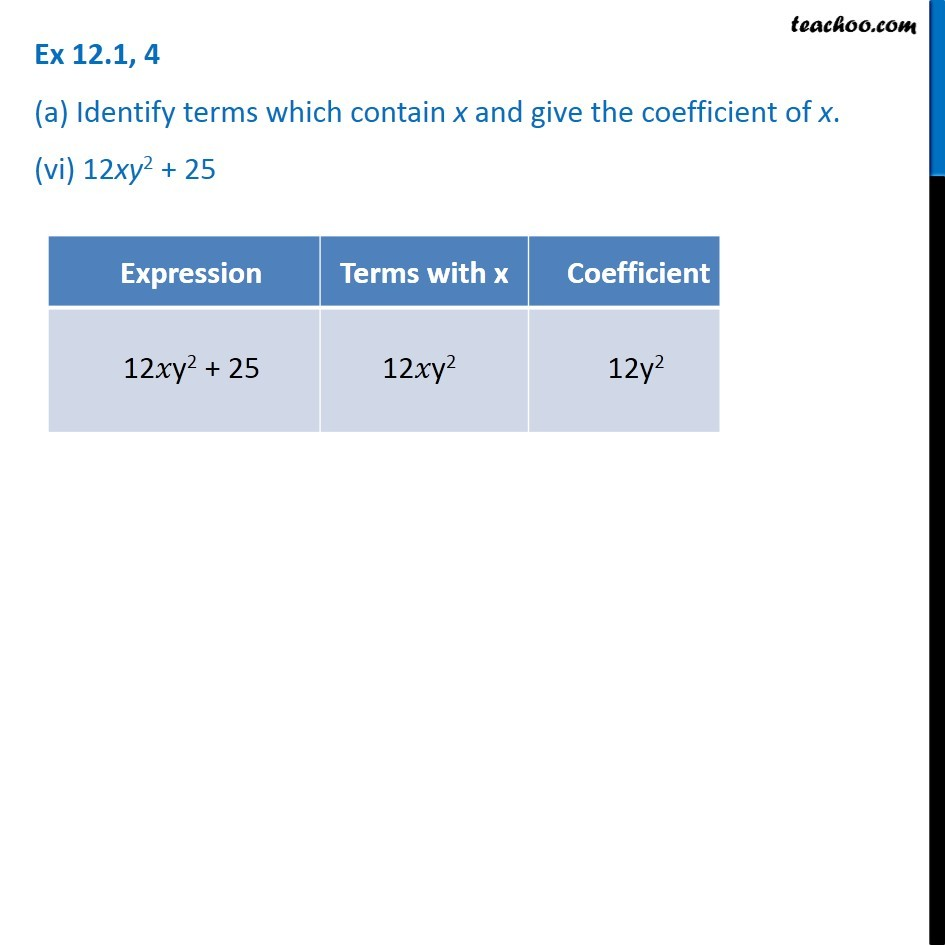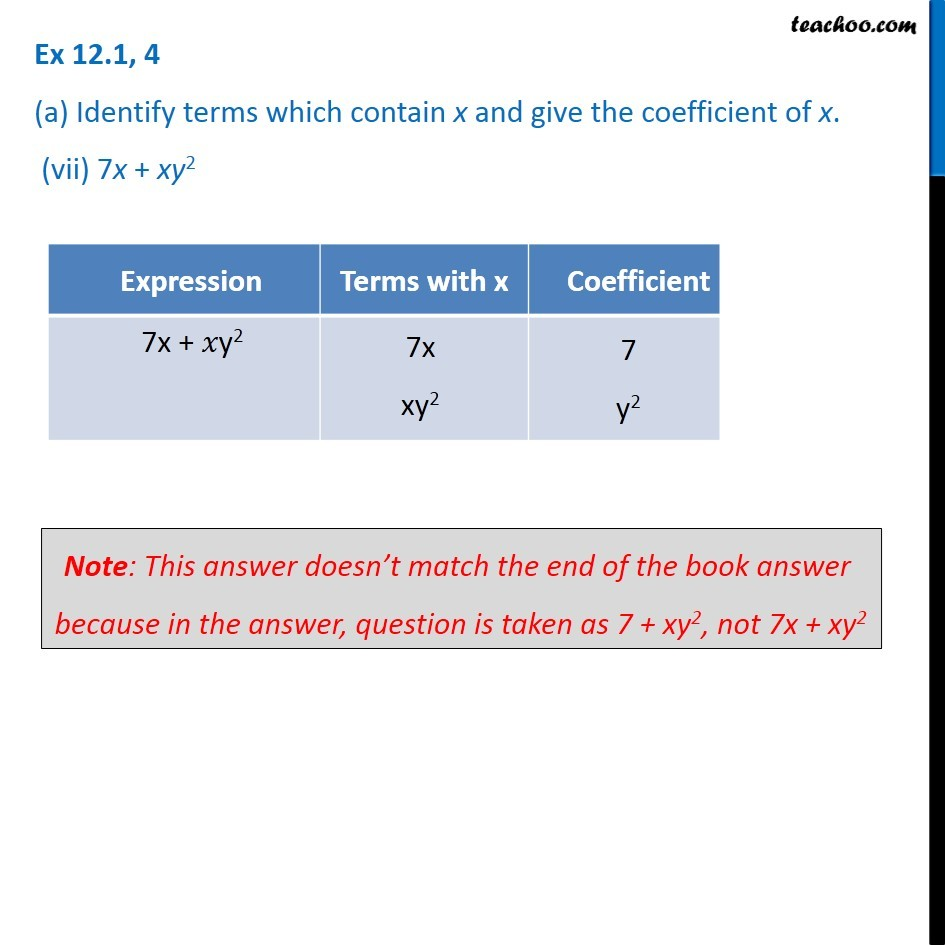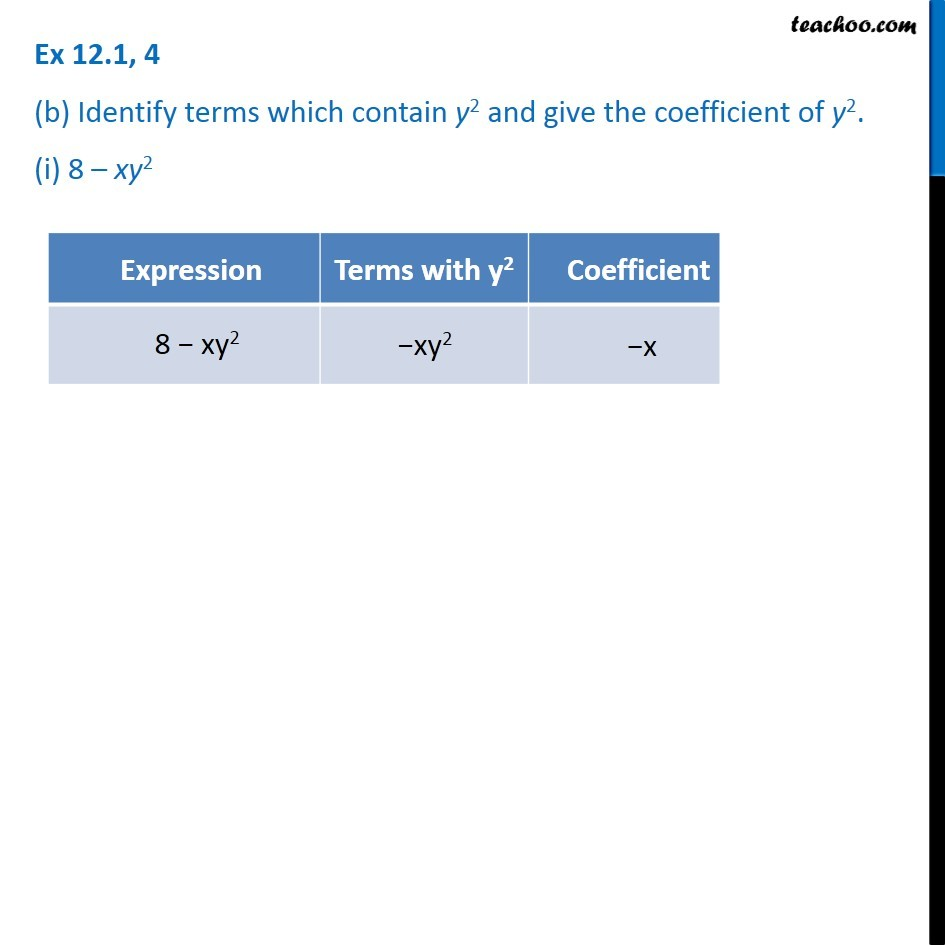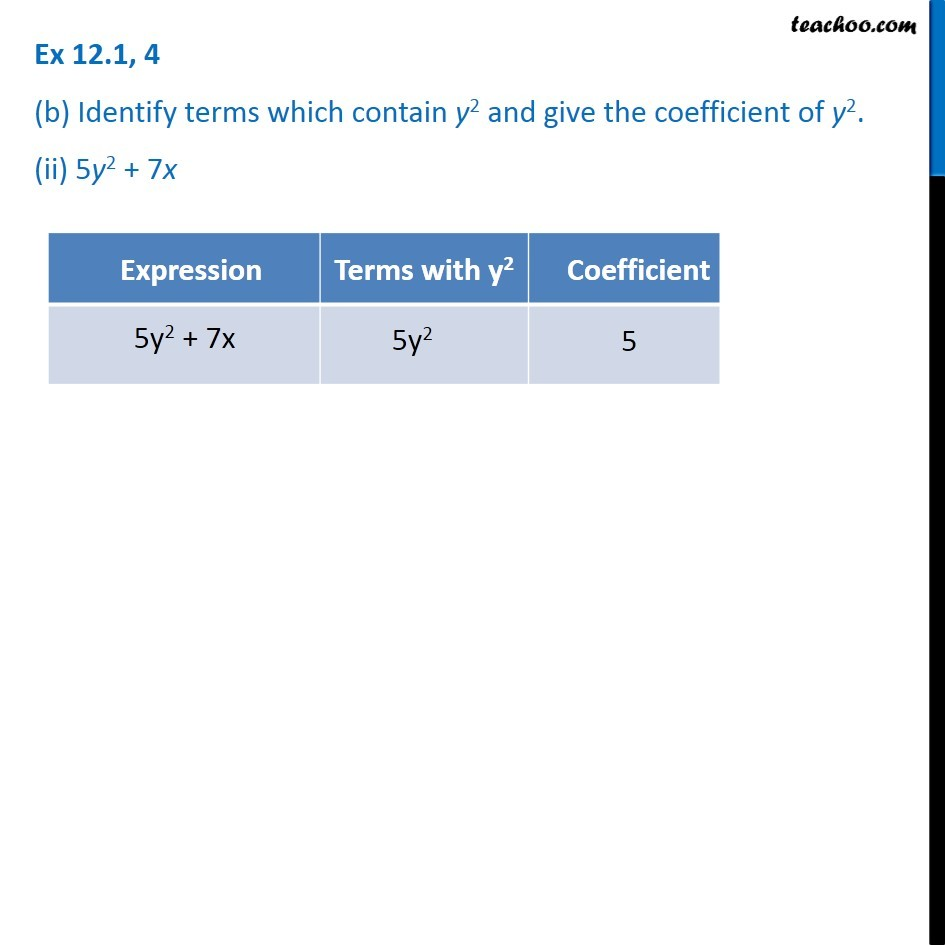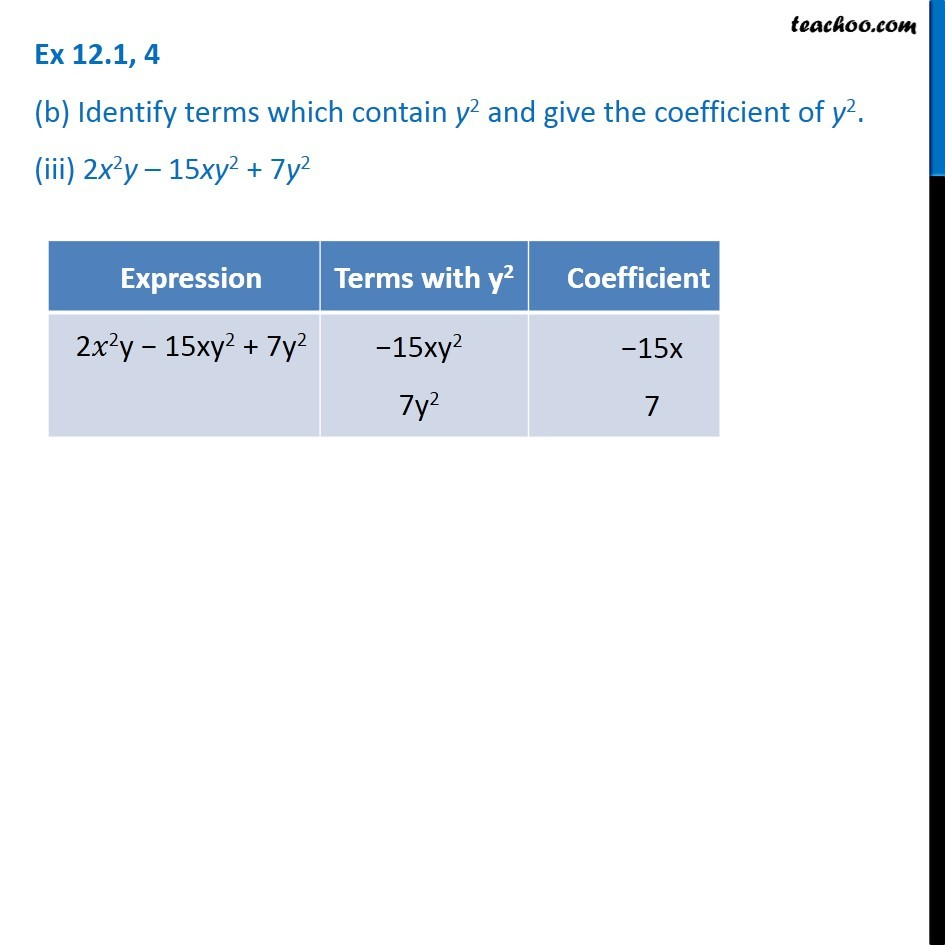Subscribe to our Youtube Channel - https://you.tube/teachoo

1. Chapter 12 Class 7 Algebraic Expressions
2. Serial order wise
3. Ex 12.1

Transcript

Ex 12.1, 4 (a) Identify terms which contain x and give the coefficient of x. (i) y2x + y Ex 12.1, 4 (a) Identify terms which contain x and give the coefficient of x. (ii) 13y2 – 8yx Ex 12.1, 4 (a) Identify terms which contain x and give the coefficient of x. (iii) x + y + 2 Ex 12.1, 4 (a) Identify terms which contain x and give the coefficient of x. (iv) 5 + z + zx Ex 12.1, 4 (a) Identify terms which contain x and give the coefficient of x. (v) 1 + x + xy Ex 12.1, 4 (a) Identify terms which contain x and give the coefficient of x. (vi) 12xy2 + 25 Ex 12.1, 4 (a) Identify terms which contain x and give the coefficient of x. (vii) 7x + xy2 Note: This answer doesn’t match the end of the book answer because in the answer, question is taken as 7 + xy2, not 7x + xy2

Ex 12.1